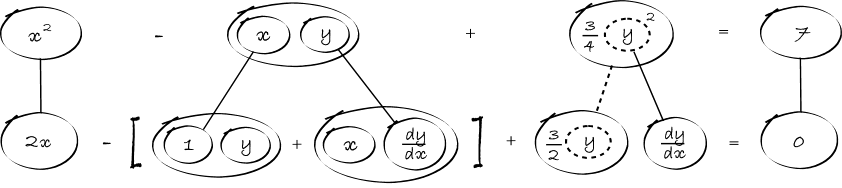# Thread: find # of points where a curve has a horizontal tangent line

1. ## find # of points where a curve has a horizontal tangent line

I am to find the number of points (by hand) where the curve x^2 - xy + (3/4)y^2 = 7 has horizontal tangent lines.

what i did was i took the derivative:

2x-y+(3/2)y = 0

simplified:

2x - (1/2) y = 0

solved for y:

2x = (1/2)y
4x = y

plugged it into the original to find my x value

x=+/- sqrt(7)/3

what do i do from here?

2.Originally Posted by Synth3t1cI am to find the number of points (by hand) where the curve x^2 - xy + (3/4)y^2 = 7 has horizontal tangent lines.

what i did was i took the derivative:

2x-y+(3/2)y = 0
Follow the chain rule for the y term, so that's (3/2)y as you say but then times dy/dx.

Use the product rule for the xy - so that'll be x times the derivative of y (for which you'll just have to write dy/dx), plus (or minus) y times the derivative of x (which is the part you did).
Then solve for dy/dx...

3.As usual, straight continuous lines differentiate (downward) with respect to x, straight dashed lines with respect to the dashed balloon expression. So the left triangular network is a 'product-rule shape' and the other one a 'chain-rule shape'.

Don't integrate - balloontegrate! Balloon Calculus: worked examples from past papers

#### Search Tags

curve, horizontal, line, points, tangent